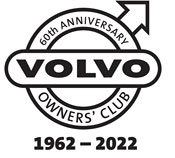# Volvo 240 242 244 245 260 262 264 265 VIN Plates

1800  |  200  |  300  |  400  |  700  |  850 - 900  |  40/50  |  60  |  70  |  80  |  90  |  XC90

This information will help you to understand the information on the VIN plate of your Volvo car.

Click on the model series number above

Example VIN Number:

YV1244842M1123456

YV1 244 84 2 M 1 123456
Manufacturer Code Vehicle Type Engine Type Gearbox Model Year Factory Code Chassis Number

Manufacturer Code
YV1 = cars YV2 = trucks YV3 = buses YV4 = multipurpose 4V1 = trucks 4V2 = 4V3 =
4V4 = trucks 4V5 = trucks 4V6 = 4VL = 4VM = 4VZ = MHA = PT. Central Sole Agency

Engine Type
11 B17A 21 = B19A 24 = B19E 26 = B19ET 37 = D20 41 = B21A 44 = B21E 45 =B21F 46 = B21ET 47 = B21FT 48 = B21LH Jet 49 = B21F MPG (1981), B21F Kjet (1982) 61 = B27A
62 = B28A 64 = B27E 65 = B27F 68 = B28E 69 = B28F 74 = D24 TIC with EGR 75 = D24 TIC without EGR 76 = D24T 77 = D24 (1981-1984) 80 = B230G 81 = B23A 82 = B230GT & B230FD 83 = B230FD w/ pulsair
84 = B23E 85 = B230FB 86 = B230FT - EGR 87 = B230FT - non EGR 88 = B23F (1983-1984), B230F (1985-95) 89 = B234F 92 = B6244F 93 =B6254F 95 = B6304F with air pump 96 = B6204F without air pump 98 = N6304G 99 = B6254G ---

Gearbox
1 = M45 2 = M46 3 = M47 6 = AW 55 7 = AW 70/71 8 = BW 55

Model Year From 1980
A = 1980 B = 1981 C = 1982 D = 1983 E = 1984 F = 1985 G = 1986 H = 1987 J = 1988 K = 1989 L = 1990 M = 1991
N = 1992 P = 1993 R = 1994 S = 1995 T = 1996 V = 1997 W = 1998 X = 1999 Y = 2000 1 = 2001 2 = 2002 3 = 2003
4 = 2004 5 = 2005 6 = 2006 7 = 2007 8 = 2008 9 = 2009 A = 2010 (code restarted) B = 2011 C = 2012 D = 2013 E = 2014 ---

Factory Code
0 = Sweden, Kalmar Plant 1 = Sweden, Torslanda Plant VCT 21(Volvo Torslandaverken) (Gothenburg) 2 = Belgium, Ghent Plant VCG 22 3 = Canada, Hatdfax Plant 4 = Italy, - Bertone models 240 5 = Malaysia, 6 = Australia,
7 = Indonesia, A = Sweden, Uddevalla Plant (Volvo Cars/TWR (Tom Walkinshaw Racing)) B = Italy, - Bertone Chongq 31 D = Italy, - Bertone models 780 E = Singapore, F = The Netherlands, Born Plant (NEDCAR) J = Sweden, Uddevalla Plant VCU 38 (Volvo Cars/ Pininfarina Sverige AB)

Engine VIN
01 = B18K 02 = B18KP 03 = B18KPD 04 = B18ES 05 = B18ED 06 = B18FTM 07 = B18KD 10 = B18U (M)-200/203 11 = B17A 12 = B18U (M)-103/113
14 = B18E/EP 15 = B18U -200/203 16 = B18U -103/113 17 = B16F 18 = B18F/FP 19 = B18FT 20 = B200 K/O 21 = B19A 22 = B200 E/O 23 = 200 E/O
24 = B19E 25 = B200 E/A 26 = B19ET 27 = B200F 28 = B200 E/E 31 = B20F 32 = B20F (M) 37 = D20 41 = B21A 44 = B21E
45 = B21F 46 = B21ET 47 = B21FT 48 = B21LH Jet 49 = B21F MPG (1981), B21F Kjet (1982) 53 = B14.4E 54 = B14.40 55 = B14.4S 61 = B27A 62 = B28A
64 = B27E 65 = B27F 68 = B28E 69 = B28F 70 = D19T (EGR) 72 = D16 74 = D24 TIC with EGR 75 = D24 TIC without EGR 76 = D24T 77 = D24 (1981-1984)
79 = D19T 80 = B230G 81 = B23A 82 = B230GT & B230FD 83 = B230FD w/ pulsair 84 = B23E 85 = B230FB 86 = B230FT - EGR 87 = B230FT - non EGR 88 = B23F (1983-1984), B230F (1985-95)
89 = B234F 92 = B6244F 93 = B6254F 95 = B6304F with air pump 96 = B6204F without air pump 98 = N6304G 99 = B6254G --- --- ---